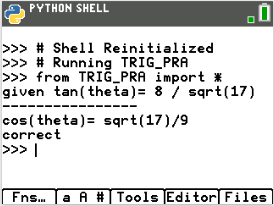# Activities

•6-8
9-12

• ##### Device
•TI-84 Plus CE Python

## Trig Ratios: Gettin' Trig-y with Python#### Activity Overview

In this coding activity for the TI-84 Plus CE Python edition, students will write a program that generates a random sine, cosine or tangent ratio.  It will ask the user to enter a different trigonometric ratio based on the given triangle information. It will evaluate the user’s input.

#### Objectives

• Use the input function and a variable to collect and store data from a user
• Use the randint function to generate triangle side lengths
• Use the randint function to determine sine, cosine, or tangent prompts
• Use if..elif..else statements to make decisions
• Use the Pythagorean Theorem to find missing triangle side lengths
• Use right triangle trigonometry ratios to solve problems